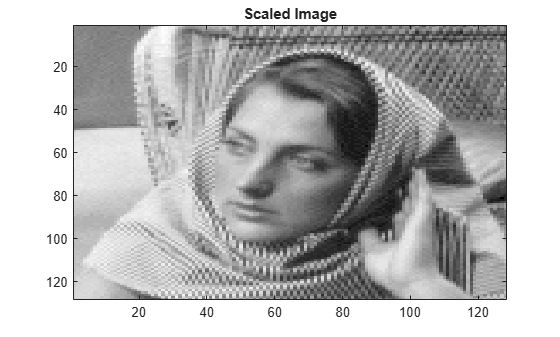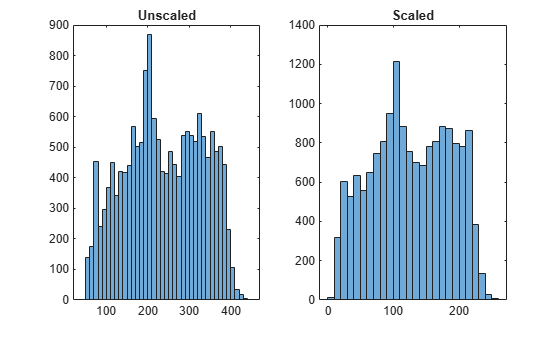# wcodemat

Extended pseudocolor matrix scaling

## Syntax

``y = wcodemat(x)``
``y = wcodemat(x,nbcodes)``
``y = wcodemat(x,nbcodes,opt)``
``y = wcodemat(x,nbcodes,opt,absol)``

## Description

`wcodemat` rescales an input matrix to a specified range for display. If the specified range is the full range of the current colormap, `wcodemat` is similar in behavior to `imagesc`.

````y = wcodemat(x)` rescales the matrix `x` as integers in the range `[1,16]`.```

example

````y = wcodemat(x,nbcodes)` rescales `x` as integers in the range `[1,nbcodes]`.```
````y = wcodemat(x,nbcodes,opt)` rescales `x` along the dimension specified by `opt`.```
````y = wcodemat(x,nbcodes,opt,absol)` rescales `X` based on the absolute values of the entries in `x` if `absol` is nonzero, or based on the signed values of `x` if `absol` is equal to zero.```

## Examples

collapse all

`load woman`

Obtain the range of the colormap.

`NBCOL = size(map,1)`
```NBCOL = 255 ```

Obtain the single-level discrete wavelet transform of the image using the Haar wavelet.

`[cA1,cH1,cV1,cD1] = dwt2(X,"db1");`

Scale the level-one approximation coefficients globally to the full range of the colormap.

`cA1scaled = wcodemat(cA1,NBCOL);`

Display the approximation coefficients without scaling and with scaling.

```image(cA1); colormap(map) title("Unscaled Image")``````image(cA1scaled) colormap(map) title("Scaled Image")```Display histograms of the unscaled and scaled approximation coefficients.

```subplot(1,2,1) histogram(cA1) title("Unscaled") subplot(1,2,2) histogram(cA1scaled) title("Scaled")```## Input Arguments

collapse all

Input, specified as a matrix.

Data Types: `double`

Upper bound of range to rescale `x` as integers, specified as a positive integer.

Data Types: `double`

Dimension along which to rescale the matrix, specified as one of the following:

• `"mat"` or `"m"` — rescale `x` globally

• `"column"` or `"c"` — rescale `x` column-wise

• `"row"` or `"r"` — rescale `x` row-wise

Data Types: `string`

Rescale basis, specified as a scalar. If `absol` is nonzero, `wcodemat` rescales `x` based on the absolute values of the elements of `x`. If `absol` is equal to zero, `wcodemat` rescales `x` based on the signed values of the elements of `x`.

Data Types: `double`